### Ecological Archives E081-027-A4

Frederick R. Adler, and Julio Mosquera. 2000. Is space necessary? Interference competition and limits to biodiversity. Ecology 81:3226-3232.

Appendix D: Conditions for existence of a two species coalition.

As in Appendix C, it can be shown that one member of any two species coalition must have mortality rate of 0. Suppose the coalition combines a fraction c0 of sites held by species 0 with c1 sites held by species z1, so that T=c0+c1. Necessary conditions for dynamic and evolutionary stability are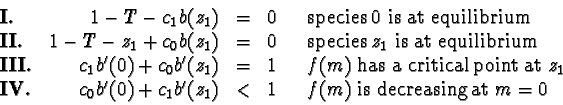The convexity assumption on b implies that b'(z1) < b'(0), which implies that c1 > c0. In any two species coalition, the species with the higher mortality rate is necessarily more common.

We write b(z)=sb0(z) where s is the slope of b at z=0 (which must be greater than 1 for such a coalition to exist). If we pick a value z1, we can try to solve for s, c0 and c1 for a given function b. Subtracting conditions I and II and simplifying gives

 s T b0(z1) = z1 (1)

Then I gives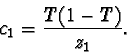(2)

From III, we can solve for T with the equation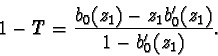(3)

Inequality IV requires that c1 > c0, or that c1/T > 1/2 or that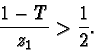Plugging in equation 3 and simplifying gives the inequality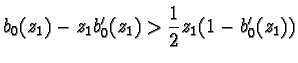z1 - 2 b0(z1) + z1 b0'(z1) < 0 . (4)

Now write b0(z)=zg(z) where g(0)=1 and g'(z)<0. Then b0'(z1) = g(z1) + z1 g'(z1), meaning that condition 4 can be rewritten

1 - g(z1) + z1 g'(z1) < 0 .

Finally, write g(z)=1-zh(z). The inequality simplifies to

h'(z1) > 0 .

For any value of z1 where h is increasing, there is a solution for s, c0 and c1 that satisfies all four conditions.

The condition h'(z1) > 0 is only a necessary condition for a stable two species coalition. To show that the coalition is indeed stable for small values of z1, and that those values correspond to values of the slope s slightly greater than 1, consider the first two terms in the series expansion of b0(z) around z=0 as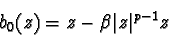for some value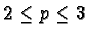. The absolute value guarantees that this function is odd. With this function,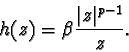If p=2, then b0(z) has a discontinuous second derivative at z=0 and the function h(z) is discontinuous at z=0. If p>2, then b0(z) has a continuous second derivative at z=0 and h(z) is increasing for small values of z.

Solving the equations I - III and discarding higher order terms gives

 T =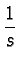z1 =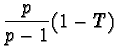c1 =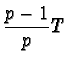c0 =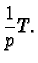(5)

With p=3, the invasion function is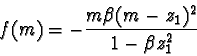which is indeed 0 for m=0 and m=z1, and negative for all other positive arguments as long as z1 is sufficiently small. With 2 < p < 3, computer algebra (Maple Version 5) indicates that invasion by intermediate species is not possible. Therefore, for values of s just greater than 1, there is a stable two species coalition described by equation 5.

[Back to E081-027]